Question

Suppose you want a telescope that would allow you to see distinguishing features as small as 3.5 km on the Moon some 384,000 km away. Assume an average wavelength of 550 nm for the light received.Required:What is the minimum diameter mirror on a telescope?

1.Explanation: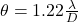And, from equation ( 2 ), we get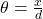Thus,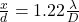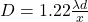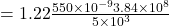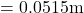Thus, the diameter of the telescope’s mirror that would allow us to see details as small as is